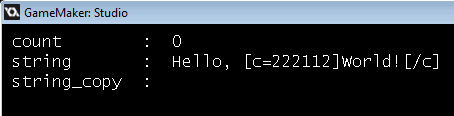# Skipping tags in a string.

#### Edwin

##### Member
Hello, nice people.

I'm trying to create a script that will skip the tags like "[color=...]", "[/c]", "[alpha=...]" and stuff and then return a script_copy().

Code:
``````/// string_copy_tag(str,index,count)

// Declare variables
var str, index, count, p, cst, cct, ast, act, et, l, cstl, cctl, astl, actl;

str = argument;
index = argument;
count = argument;

cst = '[c=';
cct = '[/c]';

ast = '[a=';
act = '[/a]';

et = ']';

l = string_length(str);

cstl = string_length(cst);
cctl = string_length(cct);

astl = string_length(ast);
actl = string_length(act);

p = index - 1;

// String copy
while (p < count) {
if (string_copy(str, p + 1, cstl) == cst) {
p += cstl;
p += string_pos(et, string_delete(str, 1, p));
} else if (string_copy(str, p + 1, cctl) == cct) {
p += cctl;
} else if (string_copy(str, p + 1, astl) == ast) {
p += astl;
p += string_pos(et, string_delete(str, 1, p));
} else if (string_copy(str, p + 1, actl) == act) {
p += actl;
} else {
p ++;
};
};

// Return
return string_copy(str, index, p);``````
I made this script but when I'm trying to change the count, it doesn't change the count itself. It's hard to explain but please look at this:"count" is a variable of an instance. "string" and "string_copy" are input and output string variables.

How can I increase the count to make this work?